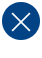# Appendix D: Example Program

```/** @file crc.h
*
* @brief Compact CRC library for embedded systems for CRC-CCITT, CRC-16, CRC-32.
*
* @par
* COPYRIGHT NOTICE: (c) 2000, 2018 Michael Barr. This software is placed in the
* public domain and may be used for any purpose. However, this notice must not
* be changed or removed. No warranty is expressed or implied by the publication
* or distribution of this source code.
*/

#ifndef CRC_H
#define CRC_H

// Compile-time selection of the desired CRC algorithm.
//
#if defined(CRC_CCITT)

#define CRC_NAME   "CRC-CCITT"
typedef uint16_t   crc_t;

#elif defined(CRC_16)

#define CRC_NAME   "CRC-16"
typedef uint16_t   crc_t;

#elif defined(CRC_32)

#define CRC_NAME   "CRC-32"
typedef uint32_t   crc_t;

#else

#error "One of CRC_CCITT, CRC_16, or CRC_32 must be #define'd."

#endif

// Public API functions provided by the Compact CRC library.
//
void    crc_init(void);
crc_t   crc_slow(uint8_t const * const p_message, int n_bytes);
crc_t   crc_fast(uint8_t const * const p_message, int n_bytes);

#endif /* CRC_H */

/*** end of file ***/
```

```/** @file crc.c
*
* @brief Compact CRC generator for embedded systems, with brute force and table-
* driven algorithm options. Supports CRC-CCITT, CRC-16, and CRC-32 standards.
*
* @par
* COPYRIGHT NOTICE: (c) 2000, 2018 Michael Barr. This software is placed in the
* public domain and may be used for any purpose. However, this notice must not
* be changed or removed. No warranty is expressed or implied by the publication
* or distribution of this source code.
*/

#include <stdint.h>

#include "crc.h"

// Algorithmic parameters based on CRC elections made in crc.h.
//
#define BITS_PER_BYTE       8
#define WIDTH               (BITS_PER_BYTE * sizeof(crc_t))
#define TOPBIT              (1 << (WIDTH - 1))

// Allocate storage for the byte-wide CRC lookup table.
//
#define CRC_TABLE_SIZE      256
static crc_t                g_crc_table[CRC_TABLE_SIZE];

// Further algorithmic configuration to support the selected CRC standard.
//
#if defined(CRC_CCITT)

#define POLYNOMIAL	       ((crc_t) 0x1021)
#define INITIAL_REMAINDER      ((crc_t) 0xFFFF)
#define FINAL_XOR_VALUE	       ((crc_t) 0x0000)
#define REFLECT_DATA(X)	       (X)
#define REFLECT_REMAINDER(X)   (X)

#elif defined(CRC_16)

#define POLYNOMIAL             ((crc_t) 0x8005)
#define INITIAL_REMAINDER      ((crc_t) 0x0000)
#define FINAL_XOR_VALUE	       ((crc_t) 0x0000)
#define REFLECT_DATA(X)	       ((uint8_t) reflect((X), BITS_PER_BYTE))
#define REFLECT_REMAINDER(X)   ((crc_t) reflect((X), WIDTH))

#elif defined(CRC_32)

#define POLYNOMIAL             ((crc_t) 0x04C11DB7)
#define INITIAL_REMAINDER      ((crc_t) 0xFFFFFFFF)
#define FINAL_XOR_VALUE        ((crc_t) 0xFFFFFFFF)
#define REFLECT_DATA(X)	       ((uint8_t) reflect((X), BITS_PER_BYTE))
#define REFLECT_REMAINDER(X)   ((crc_t) reflect((X), WIDTH))

#endif

/*!
* @brief Compute the reflection of a set of data bits around its center.
* @param[in] data The data bits to be reflected.
* @param[in] num2 The number of bits.
* @return The reflected data.
* /
static uint32_t
reflect (uint32_t data, uint8_t n_bits)
{
uint32_t   reflection = 0x00000000;

// NOTE: For efficiency sake, n_bits is not verified to be <= 32.

// Reflect the data about the center bit.
//
for (uint8_t bit = 0; bit < n_bits; ++bit)
{
// If the LSB bit is set, set the reflection of it.
//
if (data & 0x01)
{
reflection |= (1 << ((n_bits - 1) - bit));
}

data = (data >> 1);
}

return (reflection);

}  /* reflect() */

/*!
* @brief Initialize the lookup table for byte-by-byte CRC acceleration.
*
* @par
* This function must be run before crc_fast() or the table stored in ROM.
*/
void
crc_init (void)
{
// Compute the remainder of each possible dividend.
//
for (crc_t dividend = 0; dividend < CRC_TABLE_SIZE; ++dividend)
{
//
crc_t remainder = dividend << (WIDTH - BITS_PER_BYTE);

// Perform modulo-2 division, a bit at a time.
//
for (int bit = BITS_PER_BYTE; bit > 0; --bit)
{
// Try to divide the current data bit.
//
if (remainder & TOPBIT)
{
remainder = (remainder << 1) ^ POLYNOMIAL;

}
else
{
remainder = (remainder << 1);
}
}

// Store the result into the table.
//
g_crc_table[dividend] = remainder;
}
}  /* crc_init() */

/*!
* @brief Compute the CRC of an array of bytes, bit-by-bit.
* @param[in] p_message	A pointer to the array of data bytes to be CRC'd.
* @param[in] n_bytes	The number of bytes in the array of data.
* @return The CRC of the array of data.
*/
crc_t
crc_slow   (uint8_t const * const p_message, int n_bytes)
{
crc_t   remainder = INITIAL_REMAINDER;

// Perform modulo-2 division, one byte at a time.
//
for (int byte = 0; byte < n_bytes; ++byte)
{
// Bring the next byte into the remainder.
//
remainder ^= (REFLECT_DATA(p_message[byte]) << (WIDTH - BITS_PER_BYTE));

// Perform modulo-2 division, one bit at a time.
//
for (int bit = BITS_PER_BYTE; bit > 0; --bit)
{
// Try to divide the current data bit.
//
if (remainder & TOPBIT)
{
remainder = (remainder << 1) ^ POLYNOMIAL;
}
else
{
remainder = (remainder << 1);
}
}
}

// The final remainder is the CRC result.
//
return (REFLECT_REMAINDER(remainder) ^ FINAL_XOR_VALUE);

}  /* crc_slow() */

/*!
* @brief Compute the CRC of an array of bytes, byte-by-byte.
* @param[in] p_message	A pointer to the array of data bytes to be CRC'd.
* @param[in] n_bytes	The number of bytes in the array of data.
* @return The CRC of the array of data.
*/
crc_t
crc_fast (uint8_t const * const p_message, int n_bytes)
{
crc_t remainder = INITIAL_REMAINDER;

// Divide the message by the polynomial, a byte at a time.
//
for (int byte = 0; byte < n_bytes; ++byte)
{
uint8_t data = REFLECT_DATA(p_message[byte]) ^
(remainder >> (WIDTH - BITS_PER_BYTE));
remainder = g_crc_table[data] ^ (remainder << BITS_PER_BYTE);
}

// The final remainder is the CRC.
//
return (REFLECT_REMAINDER(remainder) ^ FINAL_XOR_VALUE);
}  /* crc_fast() */

/*** end of file ***/
```